# 5 Soliton existence domain

We next investigate the conditions for existence of solitons. First, we need to ensure that the origin at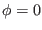is a root and a local maximum of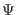in Eq. (33), i.e.,,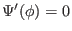and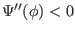at[43,44,45], where primes denote derivatives with respect to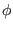. It is easily seen that the first two constraints are satisfied. We thus impose the condition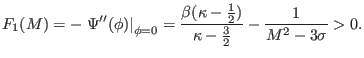(34)

Eq. (34) provides the minimum value for the Mach number,, i.e. :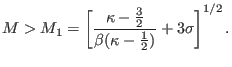(35)

Clearly,is the (normalized) electron-acoustic phase speed - cf. Eq. (16). It is thus also related to Debye screening via the screening parameterin (15), associated with the hot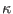-distributed electrons. We deduce that soliton solutions are super-acoustic. For Maxwellian hot electrons (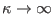) and cold cool'' electrons (), we obtain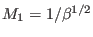, thus recovering the normalized phase speed for electron-acoustic waves in a Maxwellian plasma.  The lower Mach number limit,, increases with(via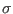), and decreases for lower values of(large excess of suprathermal electrons), and hence the sound speed in suprathermal plasmas is reduced, in comparison with Maxwellian plasmas ().

An upper limit for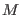is found through the fact that the cool electron density becomes complex at, and hence the largest soliton amplitude satisfies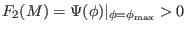. This yields the following equation for the upper limit in M: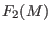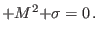(36)

Solving Eq. (36) provides the upper limit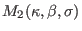for acceptable values of the Mach number for solitons to exist.

For comparison, for a Maxwellian distribution (here recovered as), the constraints reduce to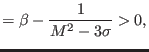(37)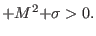(38)

The latter equation provides the upper limit, while the lower limit becomes.

In the opposite limit of ultrastrong suprathermality, i.e.,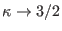, the Mach number threshold approaches a non-zero limit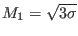, which is essentially the thermal speed, as noted above (recall that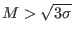by assumption). The upper limitis then given by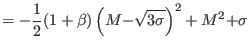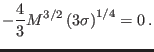(39)

Interestingly, the two limitsandboth tend to the same limit as, namely,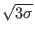, where the soliton existence region vanishes, as the kappa distribution breaks down.

We have studied the existence domain of electron-acoustic solitary waves for different values of the parameters. The results are depicted in Figs. 2-3. Solitary structures of the electrostatic potential may occur in the range, which depends on the parameters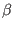,, and. We recall that we have also assumed that cool electrons are supersonic (in the sense) [43,44,45], and the hot electrons subsonic (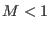), and care must be taken not to go beyond the limits of the plasma model.The intervalwhere solitons may exist is depicted in Fig. 2, in two opposite cases: in (a) and (c) two very low, and in (b) one very high value of. We thus see that for both a quasi-Maxwellian distribution and one with a large excess suprathermal component of hot electrons, bothanddecrease with an increase in the relative density parameterfor fixedand soliton speed. Further, the upper limit falls off more rapidly, and thus the existence domain in Mach number becomes narrower for higher values of the hot-to-cool electron density ratio. Comparing the two frames (a) and (b) in Fig. 2, we immediately notice that suprathermality (low) results in solitons propagating at lower Mach number values, a trend which is also seen in Fig. 2c. Another trend that is visible in Figs. 2-3a is that increased thermal pressure effects of the cool electrons, manifested through increasing, also lead to a narrowing of the Mach number range that can support solitons. Finally, we note that for, the upper limit found from Eq. (36) rises above the limitrequired by the assumptions of the model, and the latter then forms the upper limit.

Interestingly, in Figs. 2-3 the existence region appears to shrink down to nil, as the curves approach each other for highvalues. This is particularly visible in Fig. 2c, for a very low value of(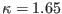). This is not an unexpected result, as high values ofare equivalent to a reduction in cool electron relative density, which leads to our model breaking down if the inertial electrons vanish. We recall that a valueis a rather abstract case, as it corresponds to a forbidden regime, since Landau damping will prevent electron-acoustic oscillations from propagating. Similarly, a high value of the temperature ratio, such as, takes us outside the physically reasonable domain. Nevertheless, as it appears that the lower and upper limits inapproach each other asymptotically for high values of, we have carried out calculations for increasing, up toforas an academic exercise, and can confirm that the two limits do not actually intersect.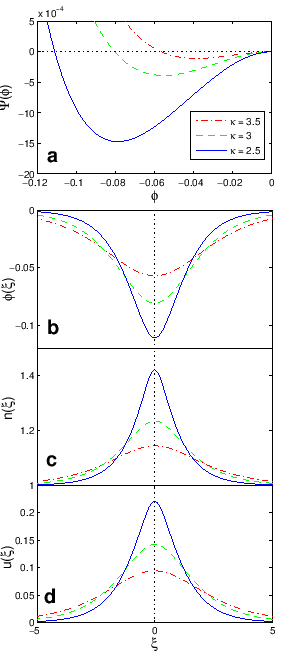Figure 3 shows the range of allowed Mach numbers as a function of, for various values of the temperature ratio. As discussed above, increasingtowards a Maxwellian distribution () broadens the Mach number range and yields higher values of Mach number. On the other hand, both upper and lower limits decrease as the limiting valueis approached. The qualitative conclusion is analogous to the trend in Fig. 2: stronger excess suprathermality leads to solitons occurring in narrower ranges of. Furthermore, as illustrated in Figs. 2 and 3a, the Mach number thresholdapproaches the upper limitfor high values ofand: both increased hot-electron density and cool-electron thermal effects shrink the permitted soliton existence region.

Figure 3b depicts the range of allowed Mach numbers as a function offor various values of the density parameter(for a fixed indicativevalue). We note that both curves decrease with an increase in. Although it lies in the damped region, we have also depicted a highregime for comparison (solid-crosses curve).

We conclude this section with a brief comparison of our work with that of Ref. . The latter did not consider existence domains at all, let alone their dependence on plasma parameters, but merely plotted some Sagdeev potentials and associated soliton potential profiles for chosen values of some of the parameters, so as to extract some trends. En passant, there is indirect mention of an upper limit in, in that it is commented that as increasing values ofare considered, at some stage solitary waves cease to exist.Ashkbiz Danehkar
2018-03-28